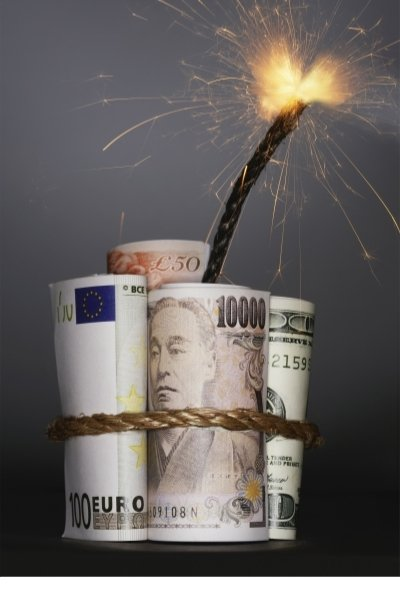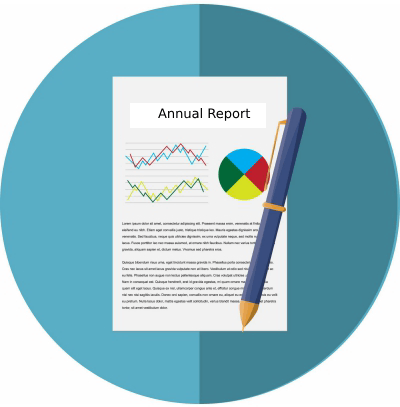10

# Return on Equity: The Hidden Leverage Time BombAnalysts love the Return on Equity (ROE) ratio and with good reason. A high ROE usually signifies a great company. Therefore, you may be tempted to use a stock screener and plug in a high number for the ROE and use the results as your list of companies to invest in.

The problem is the ROE hides information from investors who don't break it out in its true form. By using standard math, certain components cancel each other out, namely the components that would show you that a company is funding its operations through leverage.

NOTE: At the bottom of this post, there is quiz to test your knowledge on the concepts presented. Make sure you read everything on this page to ace the quiz. Everything you need is here!

## How ROE Is Calculated

This is where the time bomb comes into play and it can make a company appear to be solid when in fact the company has taken on too much debt. Most financial websites define the ROE as follows:

Return on Equity = Net Income / Equity

The formula will produce the correct result.

## The Hidden Aspect

The formula is in factored form. If you remember from your school days of learning fractions when a number in the numerator (top) is the same as a number in the denominator (bottom), with multiplication or division of the fractions, you can remove that number from both the numerator and the denominator. You can do that because any number divided by itself is 1. Any number multiplied by 1 is the number. Note, you cannot do this when you are adding and subtracting the fractions.

As an example, suppose you multiply the following three fractions:

3/4 x 5/6 x 1/3

There is a 3 in the numerator and a 3 in the denominator. This equation could be rewritten as:

3/3 x 5/6 x 1/4

The 3/3 would calculate to 1.  This means you can remove the number from the equation.

5/6 * 1/4

If you don't believe this is true, then solve all three equations. You will see they all arrive at the same result.

## The True Formula

Here is what the formula for ROE should look like:

Return on Equity = (Net Income / Sales) x (Sales / Assets) x (Assets / Equity)

You could rearrange this as follows:

Return on Equity = (Sales / Sales) x (Assets / Assets) x (Net Income / Equity)

Which Equals 1 x 1 x (Net Income / Equity)

In most cases, it's prudent to factor out terms when working with fractions. However, from a purely analytic perspective regarding using the ROE, it prevents analysts from seeing the true picture.

NOTE: Both formulas will arrive at the same result.

## The Time Bomb

When you consider the full formula, it allows you to recognize whether the Assets/Equity portion is inflated due to a company's leverage. But, this is subtle, and requires us to look at the accounting equation that defines the balance sheet:

Assets - Liabilities = Equity

This makes sense. If you applied this formula to home ownership, if you had a \$100,000 home and you put \$20,000 down, you would own \$20,000 worth of equity in the home (the bank owns the other \$80,000).

Doing the math shuffle also gives us the following formula:

Assets = Liabilities + Equity

With the same numbers from the above home ownership example, Assets = \$100,000 (what the house is worth). The liabilities is how much you were loaned for the home which is \$80,000 and the Equity = \$20,000, which was your down payment. The loan + your equity is what makes up the asset value of the home.

We can use this form of the equation to determine how much liabilities make up the Assets/Equity portion of the ROE formula. For instance, suppose a company had assets of \$80,000 and had Equity of \$20,000. What would be the liabilities in this equation? Since liabilities + equity must = assets, then liabilities would be \$60,000.

You wouldn't see easily see this breakdown from the factored equation. That's why the ROE is considered a hidden time bomb.If the debt is low, then ROE is a good measure. But, it requires more investigation.

## You Just Learned About DuPont Analysis

If you followed and understood the above analysis, then you have what it takes to understand the concept of DuPont analysis. It was created by the DuPont corporation almost 100 years ago. It helps you understand the three components of ROE which is profit margin, asset turnover, and leverage impact.It's okay to use the ROE, just make sure you do further investigation into which components of the equation have the most impact on the calculation.

## What Should the Number Read?

Assuming you have done your due diligence with the ROE, what number indicates a good ROE? The answer is the higher the better but only if the leverage factor is relatively low. The best is when the profit margin component (net income/sales) is the strongest or highest than the other components. The asset turnover can be high too. But, when the ROE is high primarily because of the leverage factor, that could indicate a problem with the company. This means that the company is increasing debt without increasing its profits or turnover or their assets.

## Example Using Microsoft

You're welcome to choose any company you like. Microsoft is a well-known company, so it's as good as any to choose.

Gather the numbers from Microsoft's website. You'll need the annual report from the Investor's Relation section. All public companies are required to publish this information.Here are the following numeric components we'll need for the DuPont analysis:

(Numbers in millions)

Sales = \$110,360 (from the Income Statement)

Net Income = \$16,571 (from the Income Statement)

Total Assets = \$258,848 (from the Balance Sheet)

Equity = \$82,718 (From the Balance Sheet)

Now, for the calculations:

Profit Margin = Net Income / Sales = \$16,571 / \$110,360 = 0.15 or 15%

Asset Turnover = Sales / Total Assets = \$110,360 / \$258,848 = 0.43 or 43%

Leverage Factor = Total Assets / Equity = \$258,848 / \$82,718 = 3.13 or 313%

Liabilities = Total Assets - Equity = \$176,130

ROE = Profit Margin x Asset Turnover x Leverage Factor = .20 or 20%

Had you calculated the ROE using the factored formula you may be seduced by the 20% ROE. On the surface, 29% is usually considered a decent number. However, as you can see from the DuPont analysis, the Leverage Factor is 313%. The Asset Turnover of 43% is not terrible but the Profit Margin is quite small when you consider the Leverage Factor is over 300%.

Breaking down the Leverage Factor further shows that the Liabilities for the company is 68% of the total assets (Liabilities / Total Assets) and the remainder of 32% is the equity. This means Microsoft is mostly using debt to finance its assets.

If you cannot see how I arrived at these numbers, always revert to the accounting equation, i.e., Assets = Liabilities + Equity. Since assets = \$258,848 and equity = \$82,718, the equity / assets is approximately 32% of assets. That means liabilities must be 68% of assets.Some investors would immediately toss this company based on this DuPont analysis. But, this could be premature. It may turn out that Microsoft has a perfectly valid reason why that Leverage Factor is as high as it is. Of course, investors may choose that is to frothy a level to feel comfortable with. But, by investigating further, they may give reasons that you find acceptable.

The whole point of this exercise is that you saw past the ROE and looked at its principle component. This gave you more insight into the ROE that would be difficult with the factored version of the calculation.

# TEST YOUR KNOWLEDGE

Take the quiz that tests your knowledge on the information provided on this page. Everything you need to answer the questions correctly is on this page. Feel free to review he page before taking the ROE quiz!

Click Here to Leave a Comment Below 10 comments